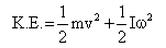Courses

# Test: Dynamics of Rotational Motion

## 10 Questions MCQ Test Physics Class 11 | Test: Dynamics of Rotational Motion

Description
This mock test of Test: Dynamics of Rotational Motion for JEE helps you for every JEE entrance exam. This contains 10 Multiple Choice Questions for JEE Test: Dynamics of Rotational Motion (mcq) to study with solutions a complete question bank. The solved questions answers in this Test: Dynamics of Rotational Motion quiz give you a good mix of easy questions and tough questions. JEE students definitely take this Test: Dynamics of Rotational Motion exercise for a better result in the exam. You can find other Test: Dynamics of Rotational Motion extra questions, long questions & short questions for JEE on EduRev as well by searching above.
QUESTION: 1

### Kinetic energy of a rolling object is the

Solution:

If an object is rolling without slipping, then its kinetic energy can be expressed as the sum of the translational kinetic energy of its center of mass plus the rotational kinetic energy about the center of mass.

QUESTION: 2

### A rotating machine is maintained at a uniform angular speed of 150 rad s-1, with a torque of 100 Nm. The power is

Solution:

Power = torque × angular velocity
P = 100 × 150
P = 15000 W
P = 15KW

QUESTION: 3

### If we apply Newton’s First Law to torque then the statement will be

Solution:

Newton's First Law for Rotation: an object at rest tends to remain at rest, and an object that is spinning tends to spin with a constant angular velocity, unless it is acted on by a nonzero net torque or there is a change in the way the object's mass is distributed.

QUESTION: 4

A torque of 20 Nm is applied to a flywheel of mass 10 kg and radius of gyration 50 cm. The resulting angular acceleration is:

Solution:
QUESTION: 5

In case of couples, which statement is not true?

Solution:

When two forces of equal magnitude opposite in direction and acting along parallel straight lines, then they are said to form a couple. The perpendicular distance between the two force forming a couple is called the arm of the couple.

‘Couple’ are the types of forces acting clockwise or anti-clockwise. We can only balance them by two forces minimum.

QUESTION: 6

A rigid body is rotating about an axis. Different particles are at different distances from the axis. Which of the following is true?

Solution:

In the fixed axis rotation we see that every point on the body has two components of velocity, one in the radial direction and one in the tangential direction. The resultant of these velocities is not the same for any two points lying in the plane of the body.
Any two points on the radial line have the radial acceleration directed towards the center of equal magnitude and the tangential acceleration of equal magnitude as well. Thus option B is correct.
All the particles lying on the curved surface of a cylinder whose axis coincides with the axis of rotation have the same speed but different velocities.

QUESTION: 7

To maximize torque on a lever, which of the following will not help?

Solution:
QUESTION: 8

Kinetic Energy of a rotating body i.e. Rotational Kinetic Energy can be converted to various forms of energy they are

Solution:

Take an example where the system is attached with spring and the spring is attached to a wall, if you rotate the system that system has KE and spring will also rotate with that system and thus spring will start to compress which will create PE in it and heat will obviously be generated.

QUESTION: 9

A 2 m rod of mass 1 kg rotates at an angular speed of 15 rad/s about its ends. Then K.E. is

Solution:

Given,
l=2m
m=1kg
ω=15rd//s
K.E.=1/2I ω
=1/2x(ml2/3) ω2
=1/2x(1x4/3)(15)2
=150J

QUESTION: 10

An object is in combined rotational and translational motion. Its K.E. is

Solution:

Total kinetic energy =